# A nuclear power station of 500 MW capacity is located at 300 km away from a load center. Select the most suitable power evacuation transmission configuration among the following options

1.

2.

3.

4.

4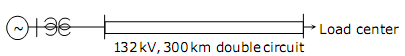Explanation :
No Explanation available for this question

# The frequency response of a linear system G (jω) is provided in the tubular form below G(jω) 1.3 1.2 1.0 0.8 0.5 0.3 G(jω) -1300 -1400 -1500 -1600 -1800 -2000

1.  6 dB and 300

2.  6 dB and -300

3.  - 6 dB and 300

4.  -6 dB and -300

4

6 dB and 300

Explanation :
No Explanation available for this question

# The steady state error of a unity feedback linear system for a unit step input is 0.1. The steady state error of the same system, for a pulse input r (t) having a magnitude of 10 and a duration of one second, as shown in the figure is

1.  0

2.  0.1

3.  1

4.  10

4

0

Explanation :
No Explanation available for this question

# Consider the following statement (i) The compensating coil of a low power factor wattmeter compensates the effect of the impedance of the current coil. (ii) The compensating coil of a low power factor wattmeter compensates the effect 0 of the impedance of the voltage coil circuit

1.  (i) is true but (ii) is false

2.  (i) is false but (ii) is true

3.  both (i) and (ii) are true

4.  both (i) and (ii) are false

4

(i) is false but (ii) is true

Explanation :
No Explanation available for this question

# A low-pass filter with a cut-off frequency of 30Hz is cascaded with a high-pass filter with a cut-off frequency of 20Hz.The resultant system of filters will function as

1.  an all-pass filter

2.  an all-stop filter

3.  an band stop (band-reject) filter

4.  a band - pass filter

4

a band - pass filter

Explanation :
No Explanation available for this question

# The CORRECT transfer characteristic is

1.

2.

3.

4.

4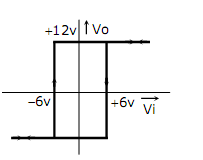Explanation :
No Explanation available for this question

# A three-phase current source inverter used for the speed control of an induction motor is to be realized using MOSFET switches as shown below. Switches S1 to S6 are identical switches   The proper configuration for realizing switches S1 to S6 is

1.

2.

3.

4.

4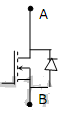Explanation :
No Explanation available for this question

# A point Z has been plotted in the complex plane, as shown in figure below. The plot of the complex number y=1/z is

1.

2.

3.

4.

4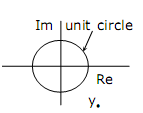Explanation :
No Explanation available for this question

# The voltage applied to a circuit is 100√2 cos (100πt) volts and the circuit draws a current of 10√2 sin (100πt +π /4) amperes. Taking the voltage as the reference phasor, the phasor representation of the current in amperes is

1.  10

2.  10

3.  10

4.  10

4

10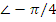Explanation :
No Explanation available for this question

1.  zero

2.  3Ω

3.  6Ω

4.  infinity

4